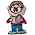## Friday, December 21, 2007

### Some More Letter Equations

I'm off for Christmas. Here's wishing you all a happy holidays and a happy new year! And I hope to see you in a week or so. If you're in the holiday spirit, why not try answering some Christmas Trivia questions.

Like yesterday, can you figure out the phrases below?
12 = D of C (12 Days of Christmas)

1001= A. N.
88= P. K.
18= H. on the G. C.
90= D. in a R. A.
200= D. for P. G. in M.
8= S. on a S. S.
3= B. M. (S. H. T. R.)
4= Q. in a G.
1= W. on a U.
5= D. in a Z. C.
57= H. V.

1.5. 1001= A. N. 1001 Arabian Nights
88= P. K. 88 piano keys
18= H. on the G. C. 18
90= D. in a R. A. 90 degrees in a right angle
200= D. for P. G. in M. 200
8= S. on a S. S. 8 sides on a stop sign
3= B. M. (S. H. T. R.) 3
4= Q. in a G. 4 quarters in a game
1= W. on a U. 1 wheel on a unicycle
5= D. in a Z. C. 5
57= H. V. 57 Heinz Varieties

2.1001 Arabian Nights
88 piano keys
18 holes on the golf course
90 degrees in a right angle
200 dollars for passing go in Monopoly
8 sides on a stop sign
3 blind mice (see how they run)
4 quarters in a game
1 wheel on a unicycle
5 digits in a zip code
57 Heinz Varieties

3.4 Quarts in a gallon

4.Does anyone know what 3=S.Y.O.A.T.O.B.G. STAND FOR?

5.http://statspuzzles.blogspot.com/2008/09/another-good-question.html

6.3 = Strikes You're Out At The Old Ball Game

7.Does any one know these
24=B F in a P G P
10=W in a I of C
2= T, D and D
41= S of W A M

8.Hello anonymous. I posted your question here:
http://statspuzzles.blogspot.com/2009/09/help-anonymous-poster-out.html

Let's see if anyone can come up with the answers.

9.Does anyone know these 180= S of S.A.
2= D of a Q
1= T in a M
2= T in a B
0= S of a H.L.
0.8= F.F. as a D
0= N of S of P.L.
4 = A.V. of N.F.

10.11.What does 20= D on the L B stand for?

12.I have this paper from school with 25 letter equations, care for a couple of them?

Leave your answer or, if you want to post a question of your own, send me an e-mail. Look in the about section to find my e-mail address. If it's new, I'll post it soon.

Please don't leave spam or 'Awesome blog, come visit mine' messages. I'll delete them soon after.

Enter your Email and join hundreds of others who get their Question of the Day sent right to their mailbox

The Lamplight Manor Puzz 3-D